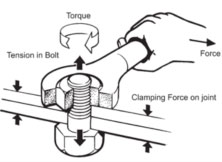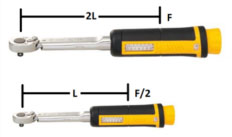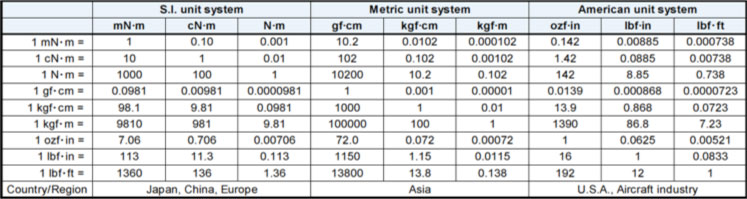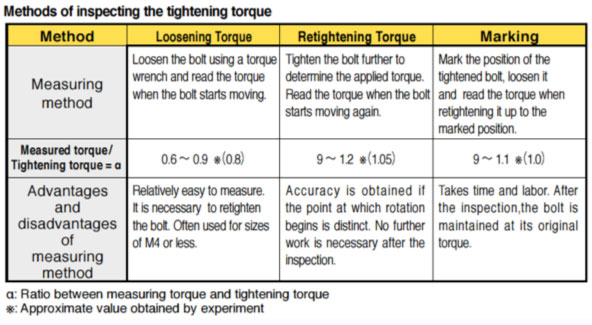# FAQ

Q. What is torque?
Torque is twisting or turning force.
Torque is used for creating Tension.
Torque is the application of a Force acting at a radial Distance and tending to cause rotation.Q. How to calculate torque?
Torque(T) is the result of multiplying the value of Force(F) applied by the Distance(L) from the point of application.
T = F x L
= 2F x L/2 (if force is doubled, halving the length will develop the same torque)
= F/2 x 2L (if force is halved, doubling the length will develop the same torque)Q. What are Torque units?
The units used to describe torque reference both a force and a length.
There are three common torque units:
• SI (International Standard) based on Newton meters.
[N.m] Newton meter
1000 [nNm]=100 [cNm]=1 [Nm]=0.001 [kNm]
• Metric based on kilogram force centimetres.
[kgf.cm] Kilogram force centimeter
[gf.cm.]=1[kgf.cm]=0.01[kgf.m]
• American/ English based on inch pounds.
[lbf.in] inch pounds
16[ozf.in]=1[lbf.in.]=0.0833[lbf.ft.]

The International Standards Organisation (ISO) recommend the use of the Newton metre (N.m) to denote the ‘base' torque unit.Q. Why is Torque important?
Fasteners tightening is done in order to stop objects from moving--to fix them. The following are the major objectives of fastener tightening:
1. For fixing and joining objects
2. For transmitting driving force and braking force
3. For sealing drain bolts, gas, and liquid

The fixing force is referred to as axial tension or tightening force and the objective of screw tightening is to apply an appropriate amount of axial tension.

Although axial tension is really what needs to be controlled and measured, it is very difficult to do so, therefore torque is used as a substitute characteristic for administering and controlling tightening operations.

Q. What are the methods of inspecting the Tightening Torque?

Estimate how much torque was applied in the screw tightening, and carry out an inspection of the tightening operation with the following methods.

• Loosening Torque method
• Retightening Torque method
• Marking methodUnichi Pte Ltd - Specialised in Torque Products © 2021